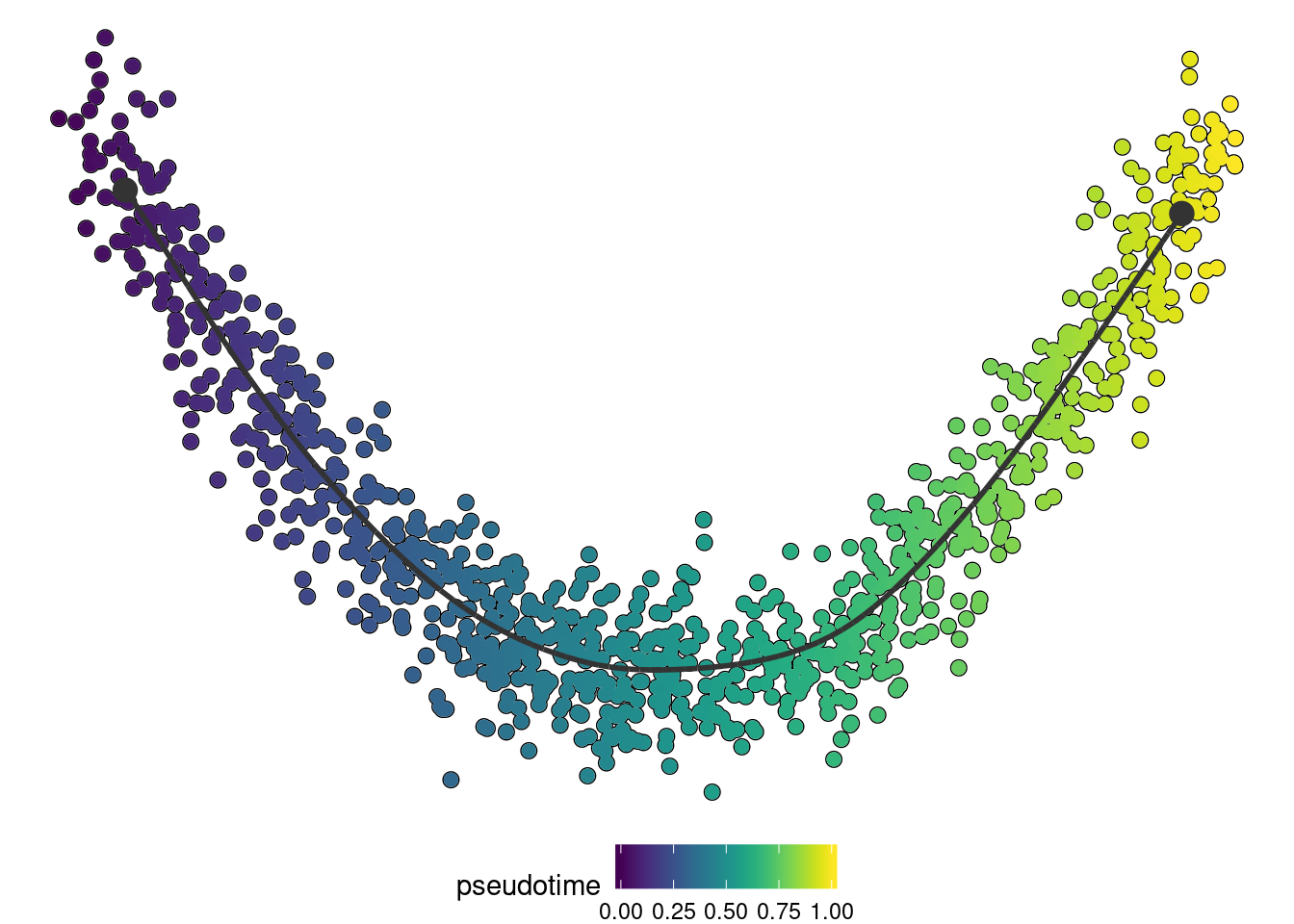# Wrapping in R

``library(dynwrap)``

This vignette describes how to include your own method into the dynverse workflow. We’ll illustrate this with a very basic method: using one of the components of a PCA as the pseudotime.

A method defined in R has two parts: a definition and a function.

## Definition

The definition contains information on the method, its parameters and its required or optional inputs. The possibilities are quite extensive (see `dynwrap::definition()`), but essentials are:

• Method definition with the id of the method
• Parameters if applicable. These are defined using the `dynparam` package.
• Required inputs (input_required) and if applicable some optional inputs (input_optional). Inputs can be:
• expression or counts
• Some prior information, see `dynwrap::priors`
``````definition <- definition(
method = def_method(
id = "comp1"
),
parameters = def_parameters(
dynparam::integer_parameter(
id = "component",
default = 1,
distribution = dynparam::uniform_distribution(1, 10),
description = "The nth component to use"
)
),
wrapper = def_wrapper(
input_required = "expression",
input_optional = "start_id"
)
)``````

## Run function

The actual inference of the trajectory is done in the run function. This function accepts:

• expression or counts
• priors A list containing the requested priors
• parameters A list containing the parameters
• seed The seed provided by the user. In R this seed is already automatically set
• verbose Integer of the level of verbosity that will be shown to the user. Based on this message, you can print out different info/warning/error messages.
``````run_fun <- function(expression, priors, parameters, seed, verbose) {
pca <- prcomp(expression)

pseudotime <- pca\$x[, parameters\$component]

# flip pseudotimes using start_id
if (!is.null(priors\$start_id)) {
if(mean(pseudotime[start_id]) > 0.5) {
pseudotime <- 1-pseudotime
}
}

dynwrap::wrap_data(cell_ids = rownames(expression)) %>%
}``````

This function returns a trajectory object as described in create a trajectory. You may also add other relevant information to this output, often some timing checkpoints (`add_timings`), dimensionality reduction (`add_dimred`) or a cell clustering (`add_grouping`). Check out the reference for an overview or post an issue or pull request if you want a type of output to be added.

## Testing it out

To wrap the method, use `create_ti_method_r` with the definition and run function. At this stage you can also define some R packages that have to be installed or loaded.

``ti_comp1 <- create_ti_method_r(definition, run_fun, package_loaded = "dplyr")``

This function will return another function that can be used to change any parameters when running the method. This function is also useful to document any parameters when included in an R package.

That’s it, let’s try it out:

``````dataset <- dynwrap::example_dataset
trajectory <- infer_trajectory(dataset, ti_comp1())``````
``````if ("dynplot" %in% rownames(installed.packages())) {
dynplot::plot_dimred(trajectory, color_cells = "pseudotime" , expression_source = as.matrix(dataset\$expression))
}``````## Making your method available for other users

The easiest way to make this method available to others is to put it in an R package which exports the `ti_*` function. Here you can also provide documentation using roxygen2. The parameters can be automatically documented from the information provided in the description using `@eval dynwrap::generate_parameter_documentation(definition)` (adapt the name of definition if necessary).

``````#' Infer a trajectory from the first principal component
#'
#' @eval dynwrap::generate_parameter_documentation(definition)
#'
#' @import dplyr
#' @export
#'
#' @examples
#' dataset <- dynwrap::example_dataset
#' model <- dynwrap::infer_trajectory(dataset, ti_comp1())
ti_comp1 <- create_ti_method_r(definition, run_fun)``````

The parameter documentation will look like follows:

``generate_parameter_documentation(definition)``
``##  "@param component The nth component to use. Domain: U(1, 10). Default: 1. Format: integer."``

We also strongly encourage you to include within our collection of TI methods available in the dynmethods R package. Feel free to create an issue or send us a pull request.